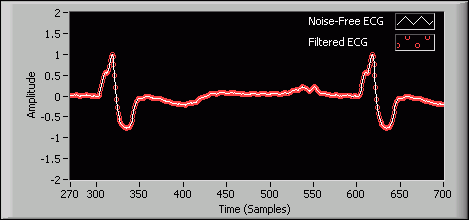# Notch and Peak Filters (Digital Filter Design Toolkit)

LabVIEW 2011 Digital Filter Design Toolkit Help

Edition Date: June 2011

Part Number: 371325F-01

»View Product InfoDownload Help (Windows Only)

Use notch filters to suppress noise at a specified frequency, such as an AC powerline frequency. Use peak filters to enhance the signal at a particular frequency. The following figure shows a typical notch filter.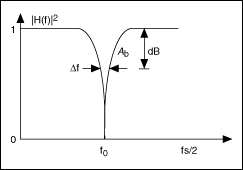In a notch filter, f 0 denotes the center frequency and Δf denotes the frequency bandwidth at Ab . The default value of Ab is −3 dB. Q = f 0f, which denotes the sharpness of the notch. Increasing the value of Q results in a sharper notch filter.

The following figure shows a typical peak filter.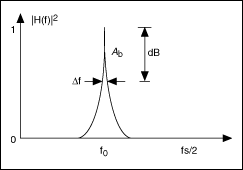In a peak filter, Q denotes the sharpness of the peak. Increasing the value of Q results in a sharper peak filter.

The DFD IIR Notch Peak Design VI designs a second-order infinite impulse response (IIR) notch or peak filter using a bilinear transform method. The following equation shows the transfer function of a notch filter.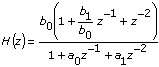The following equation shows the transfer function of a peak filter.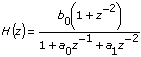where a 0, a 1, b 0, and b 1 are filter coefficients.

For example, assume a time waveform of a noisy electrocardiogram (ECG) signal sampled at 333 Hz, as shown in the following figure: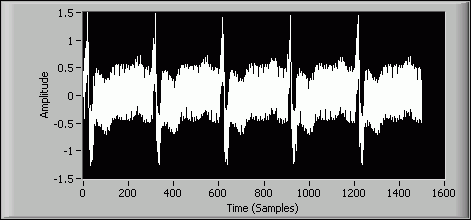The following figure shows the spectrum of the same noisy signal. In this figure, you can identify the noise at 60 Hz.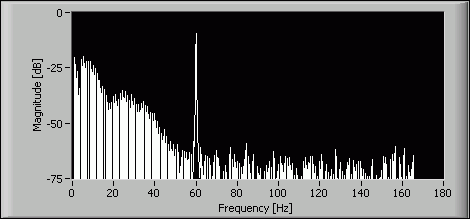To remove the noise at 60 Hz, you can design a notch filter using the DFD IIR Notch Peak Design VI with the following specifications:

DFD IIR Notch Peak Design VI Inputs Value
filter type Notch
f0 [Hz] 60
Q factor 40
fs [Hz] 333

The following figure shows the magnitude response of the resulting notch filter.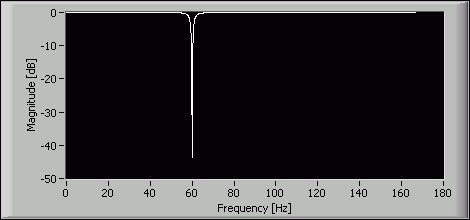The following figure shows that the filtered ECG signal is close to the original noise-free samples.